# RD Sharma Solutions for Class 9 Maths Chapter 2 Exponents of Real Numbers Exercise 2.2

RD Sharma Class 9 Mathematics Chapter 2 Exercise 2.2 Exponents of Real Numbers is provided here. This exercise is about rational exponents of real numbers and laws of rational exponents. RD Sharma Solutions Class 9 chapter 2 are provided by subject experts at BYJU’S. Click on the link to download free PDF of chapter 2 exercise 2.2.

## Download PDF of RD Sharma Solutions for Class 9 Maths Chapter 2 Exponents of Real Numbers Exercise 2.2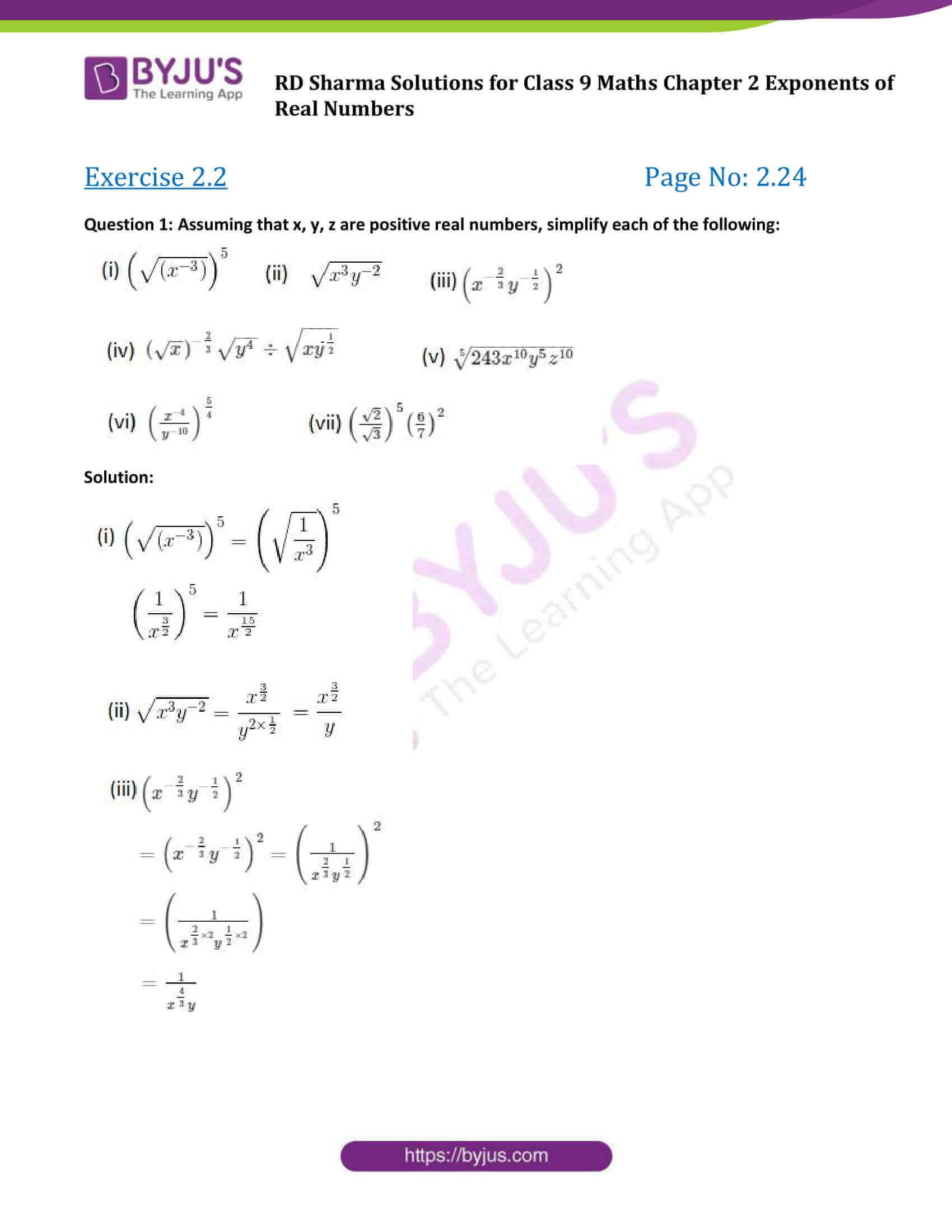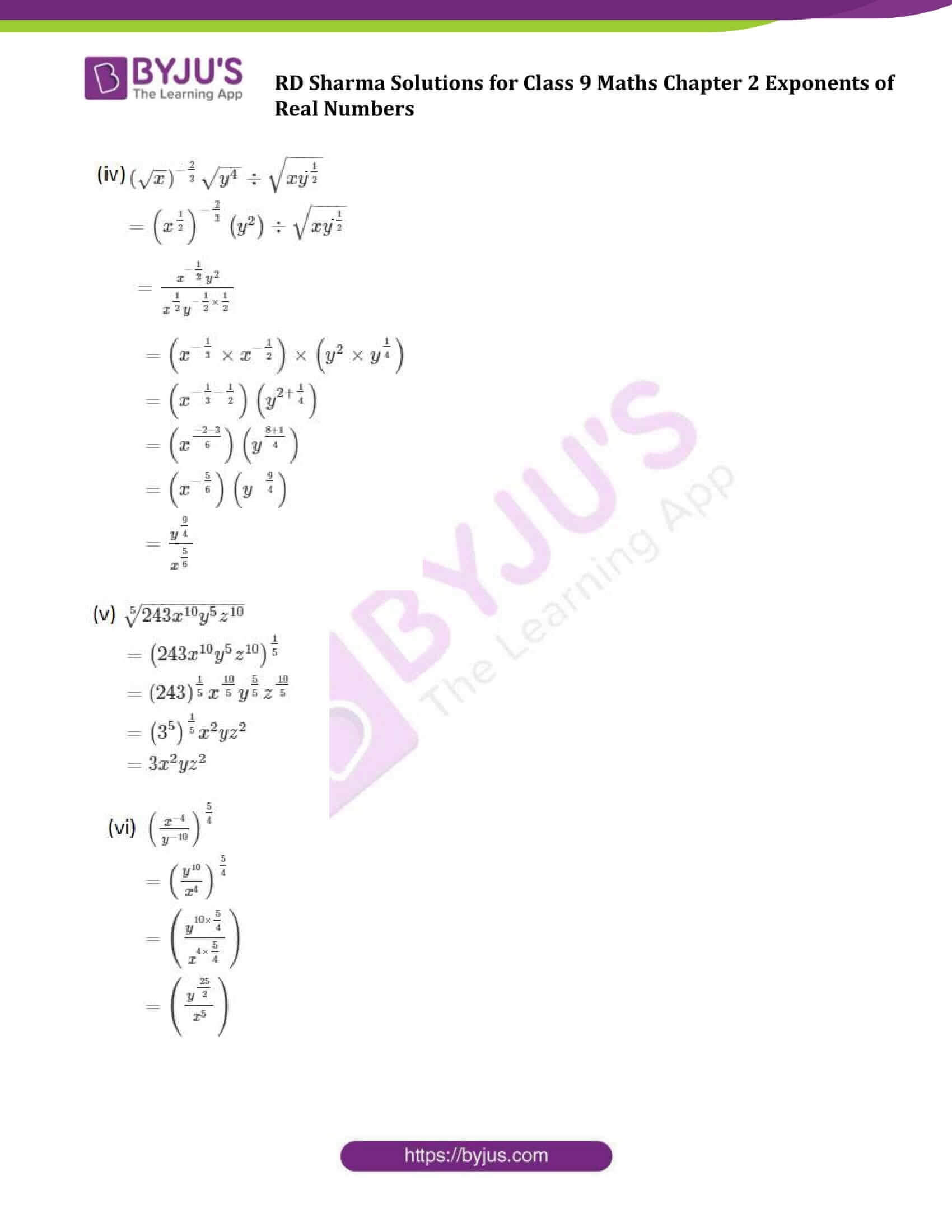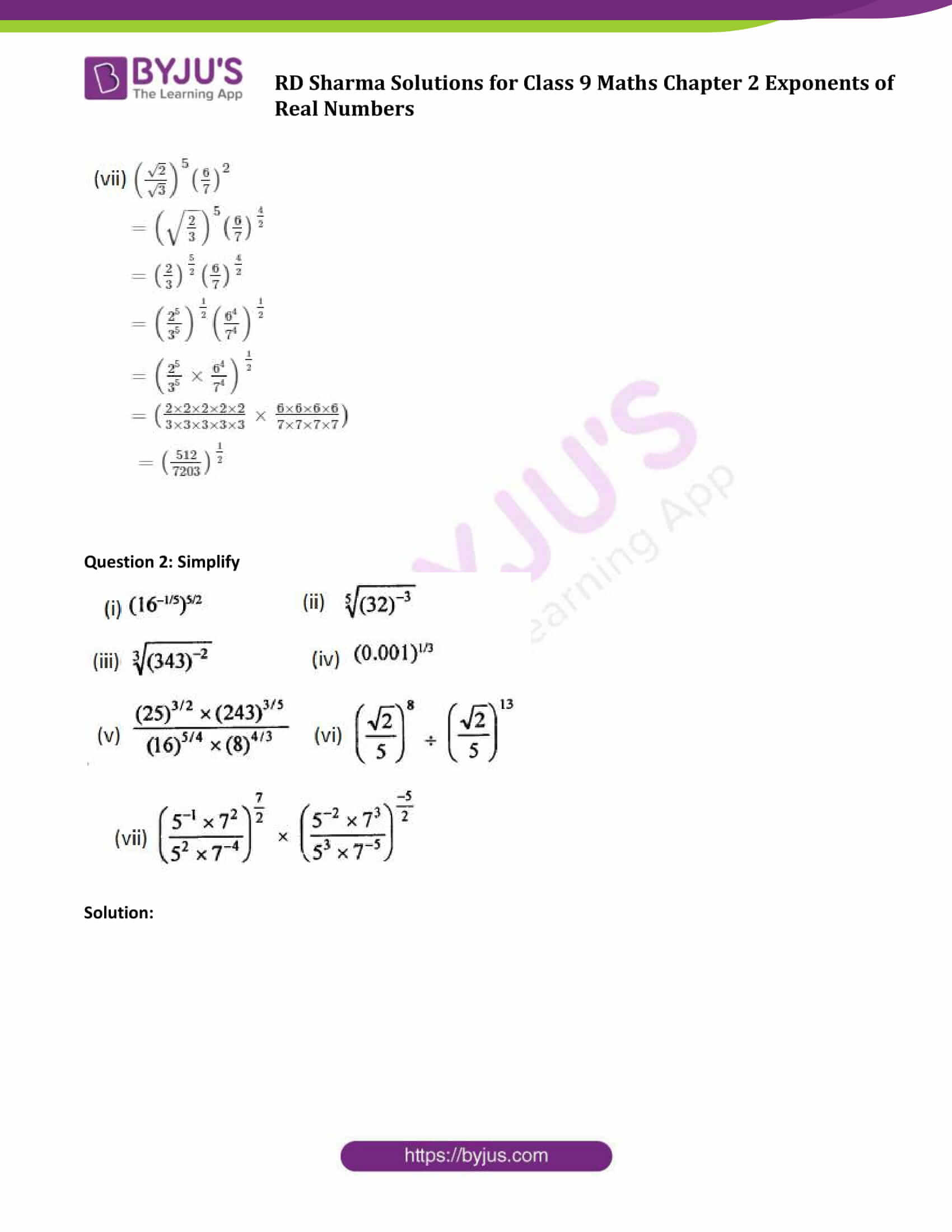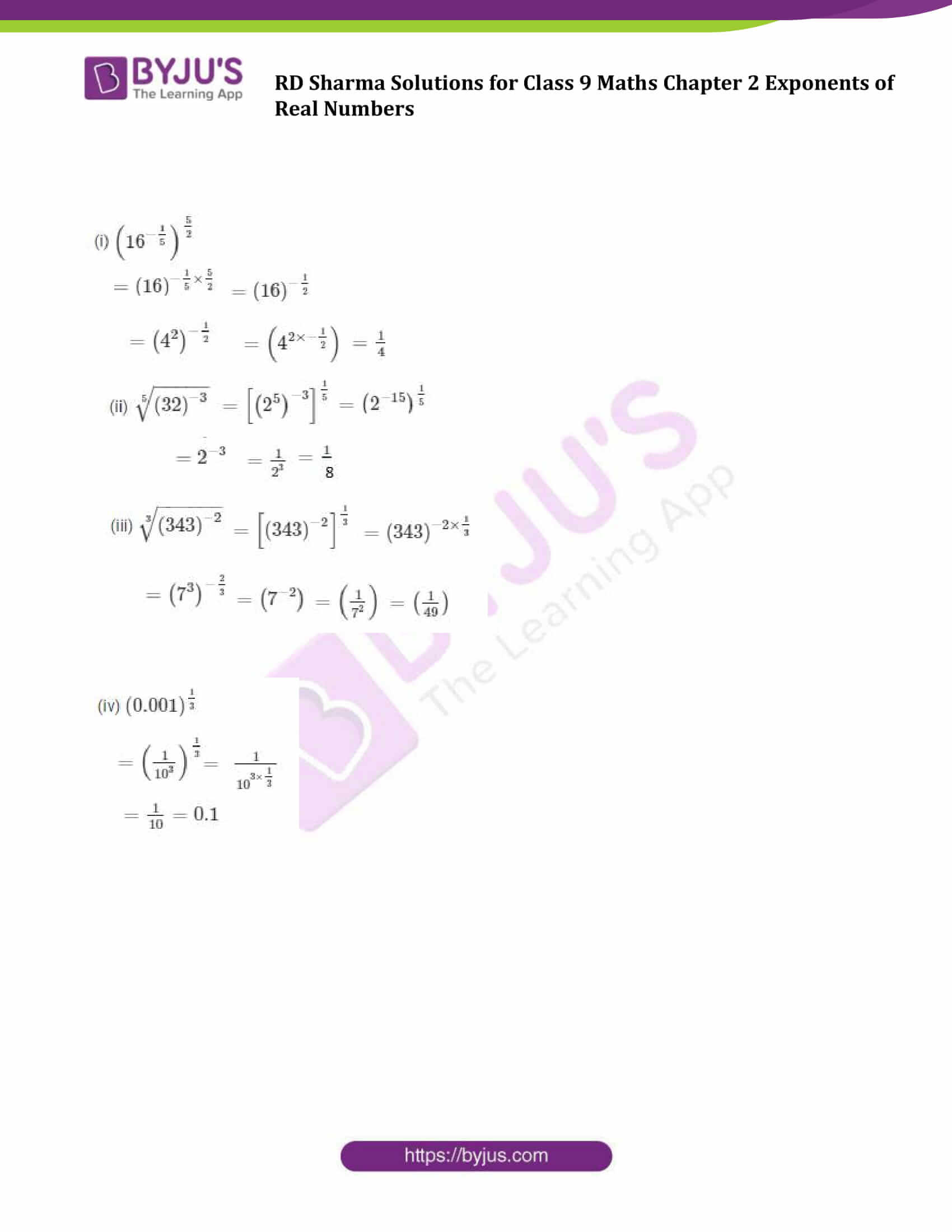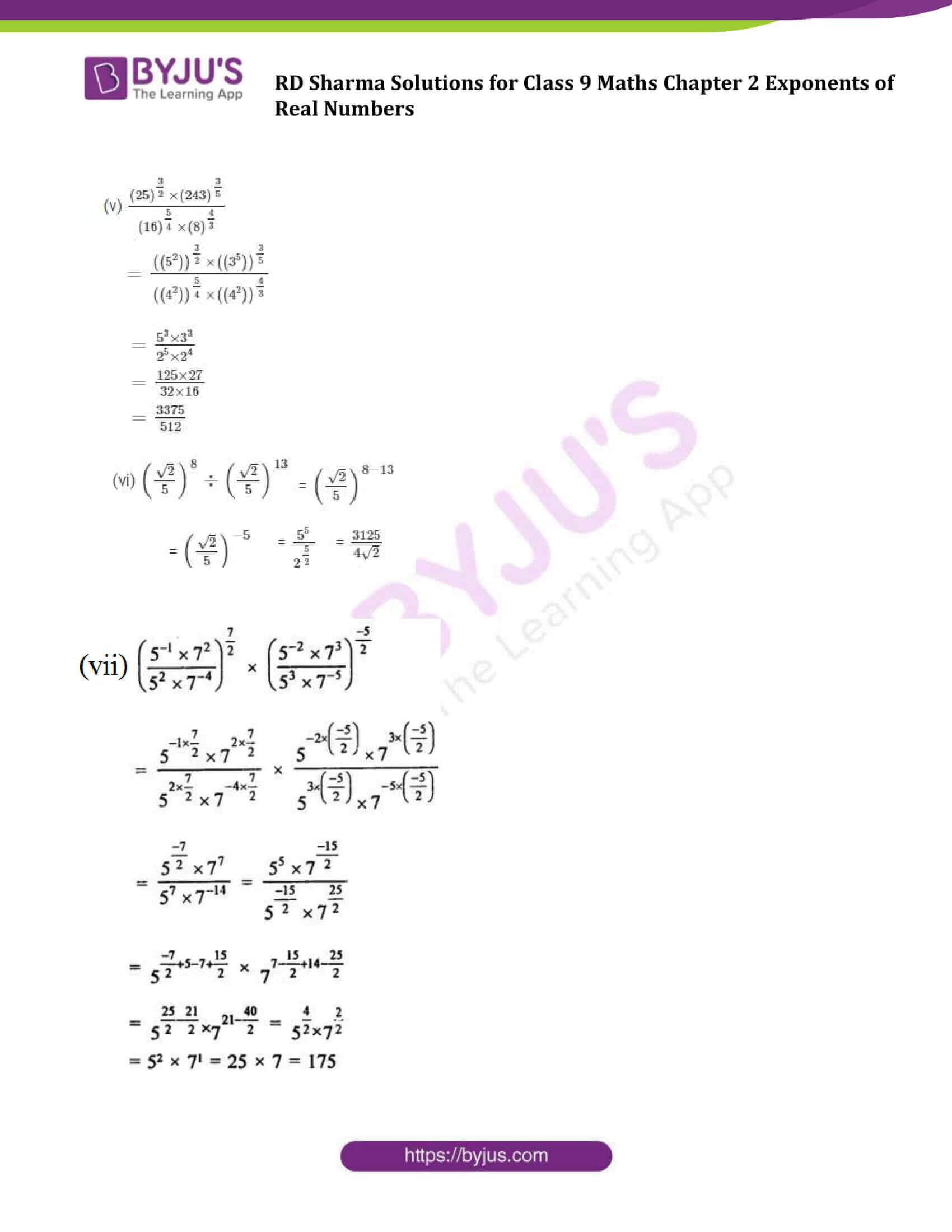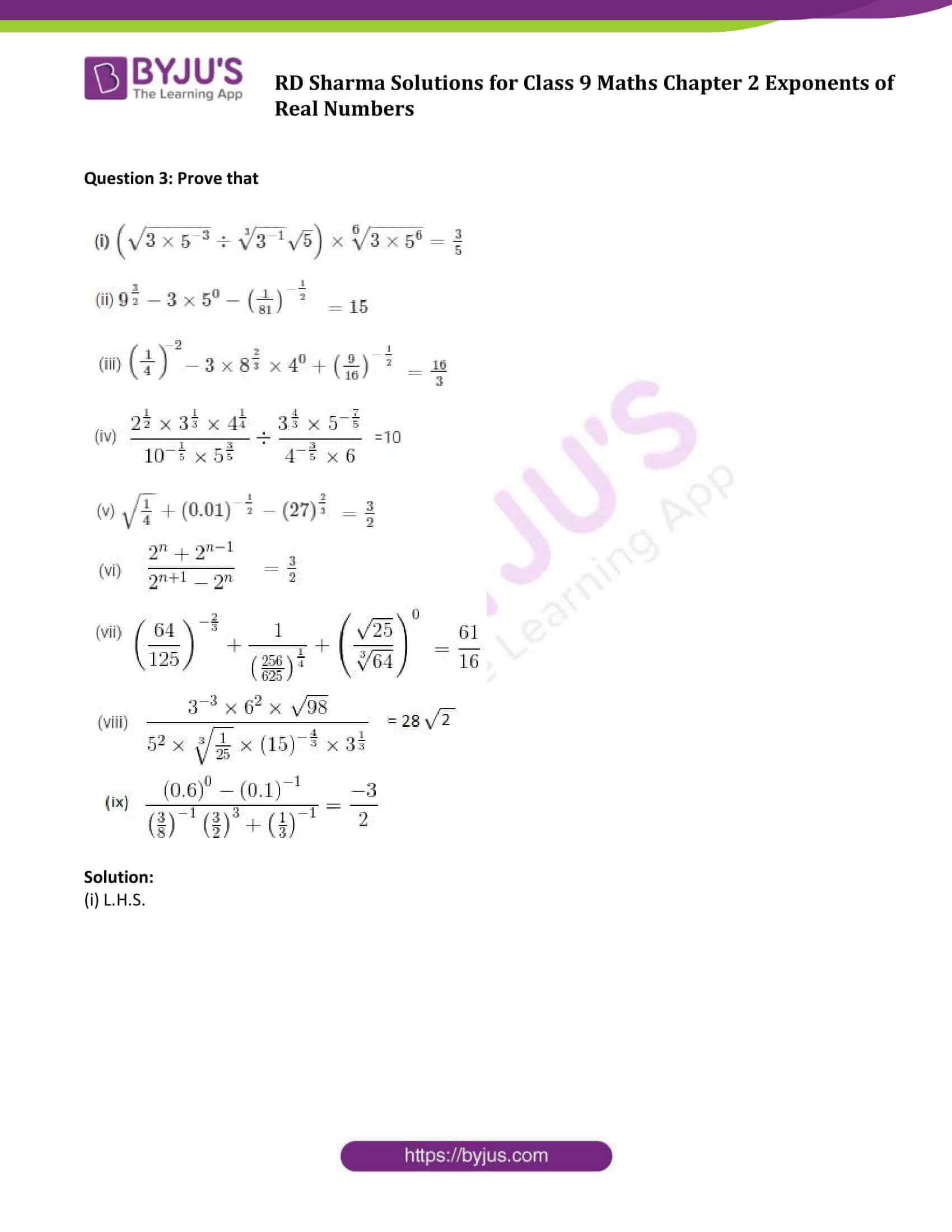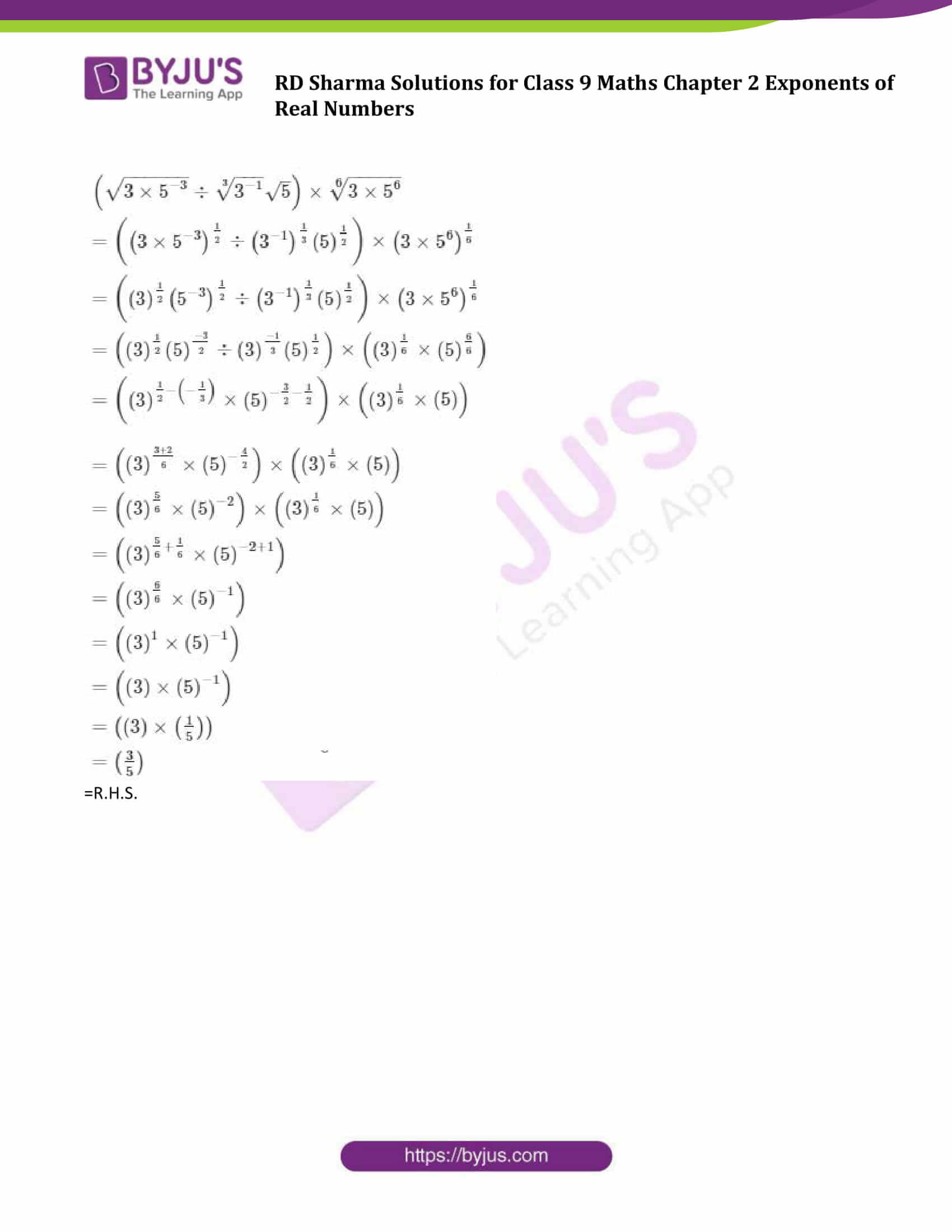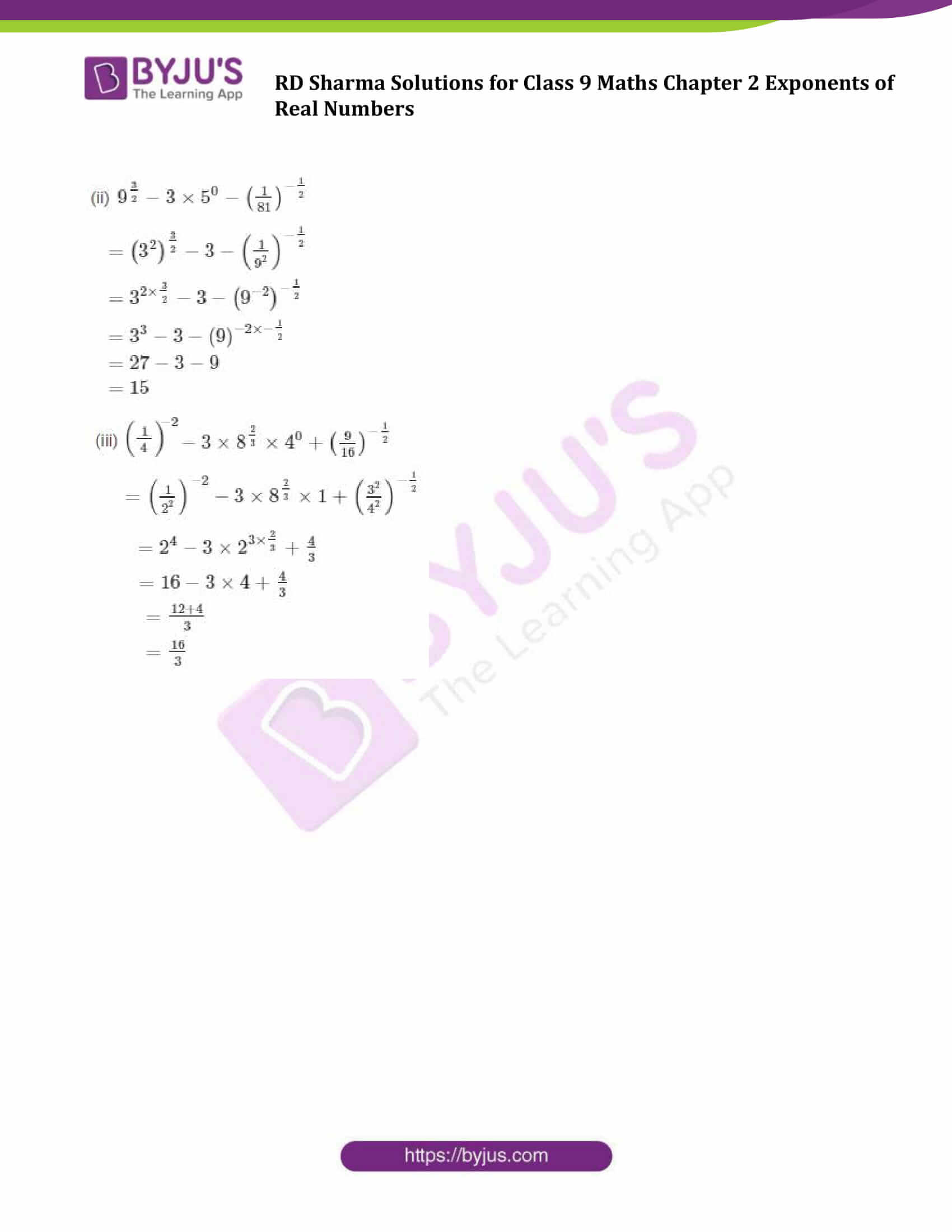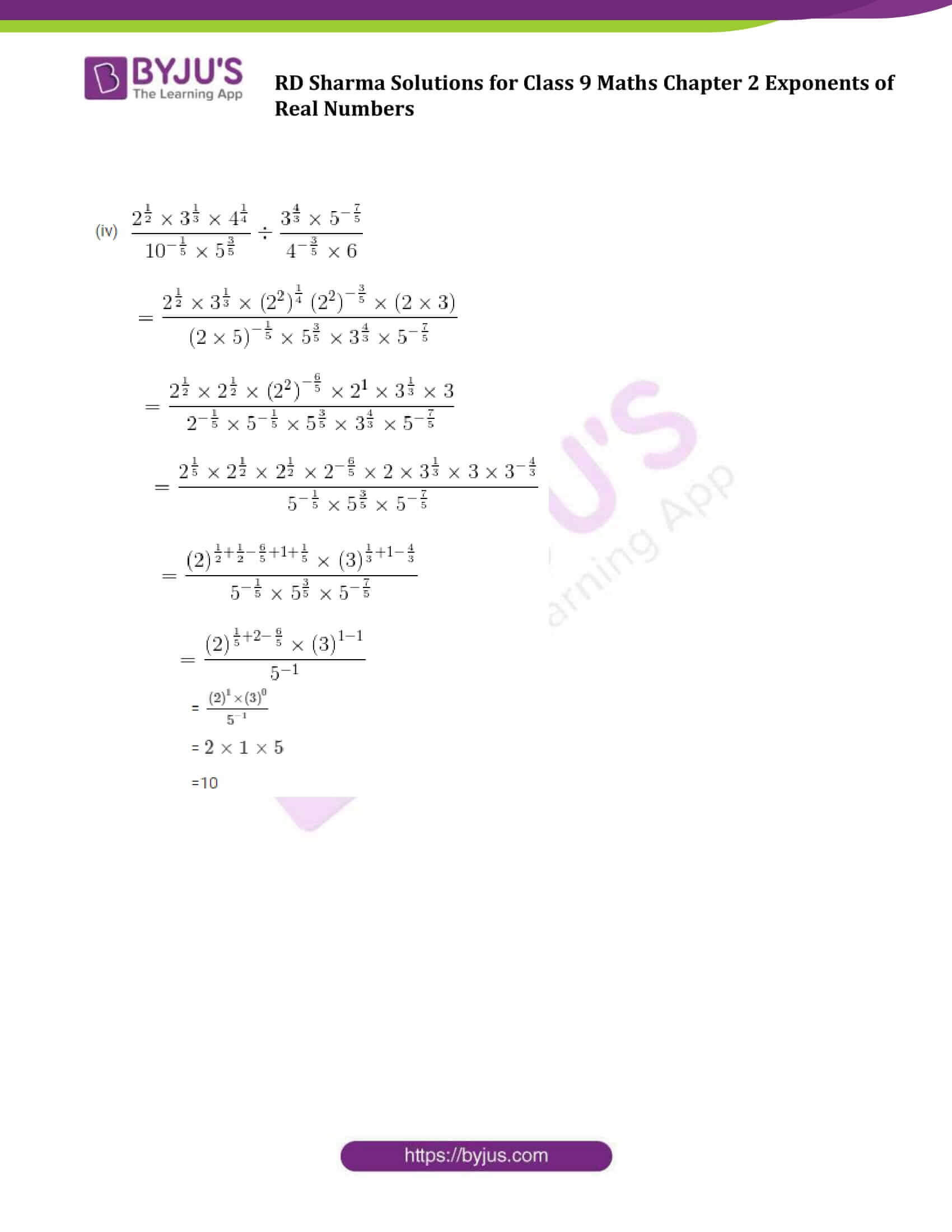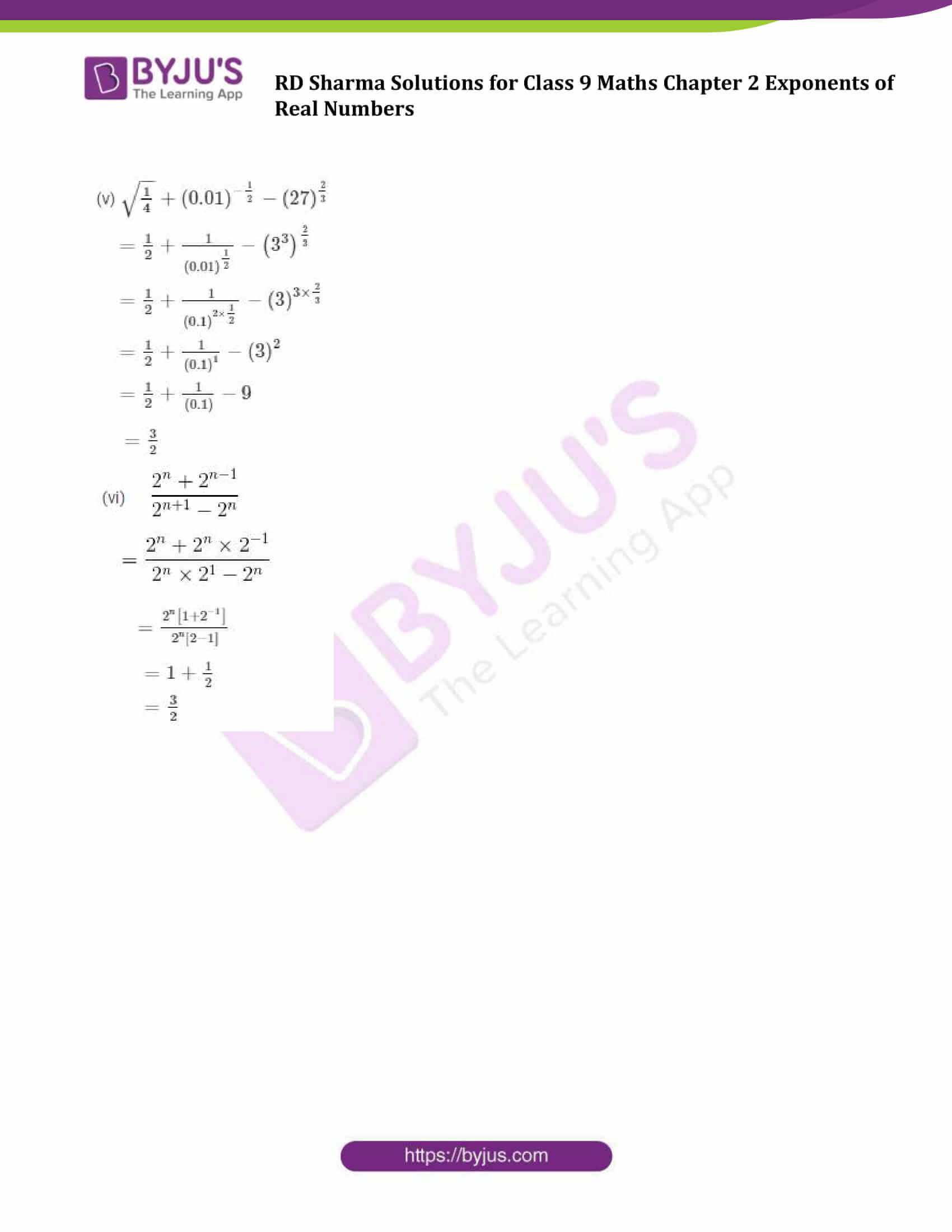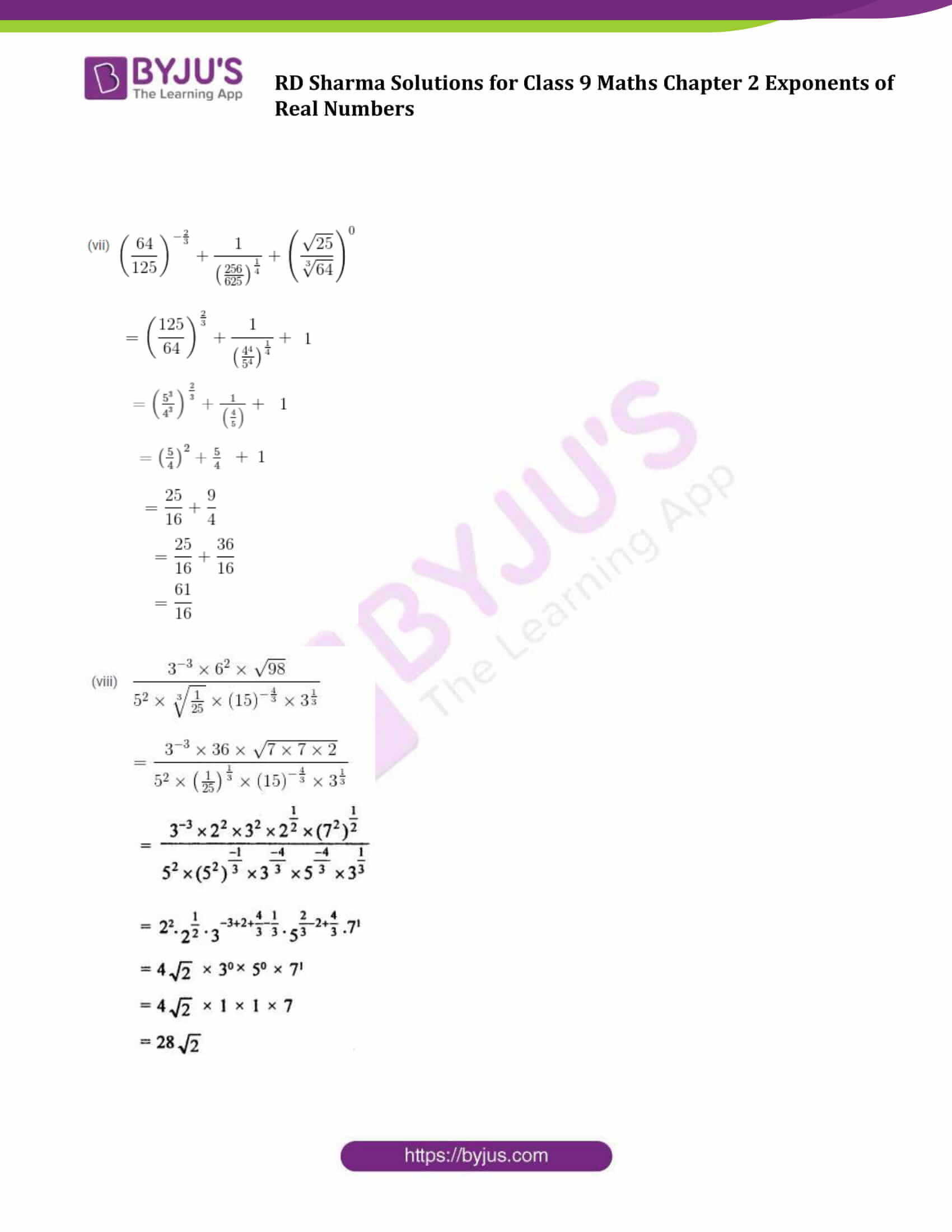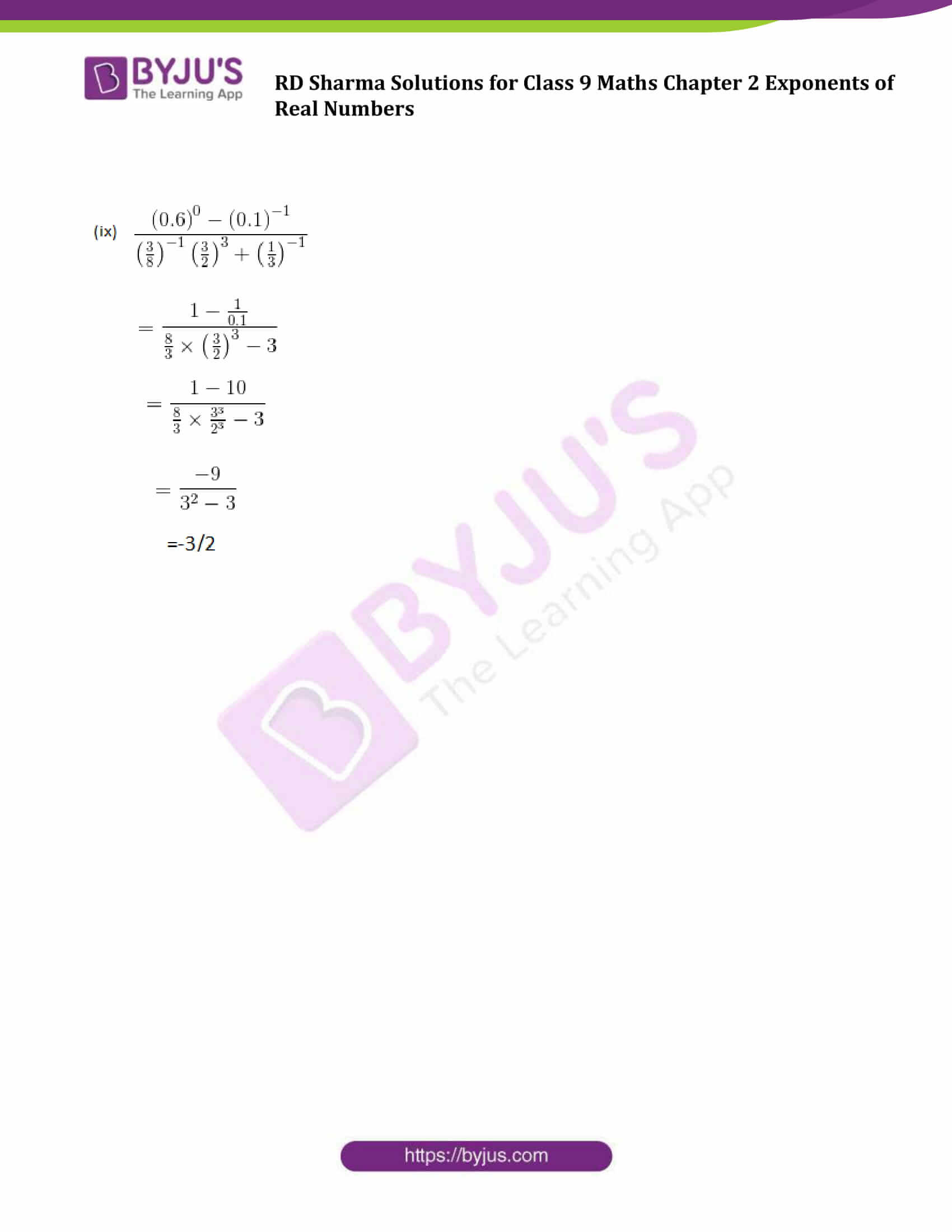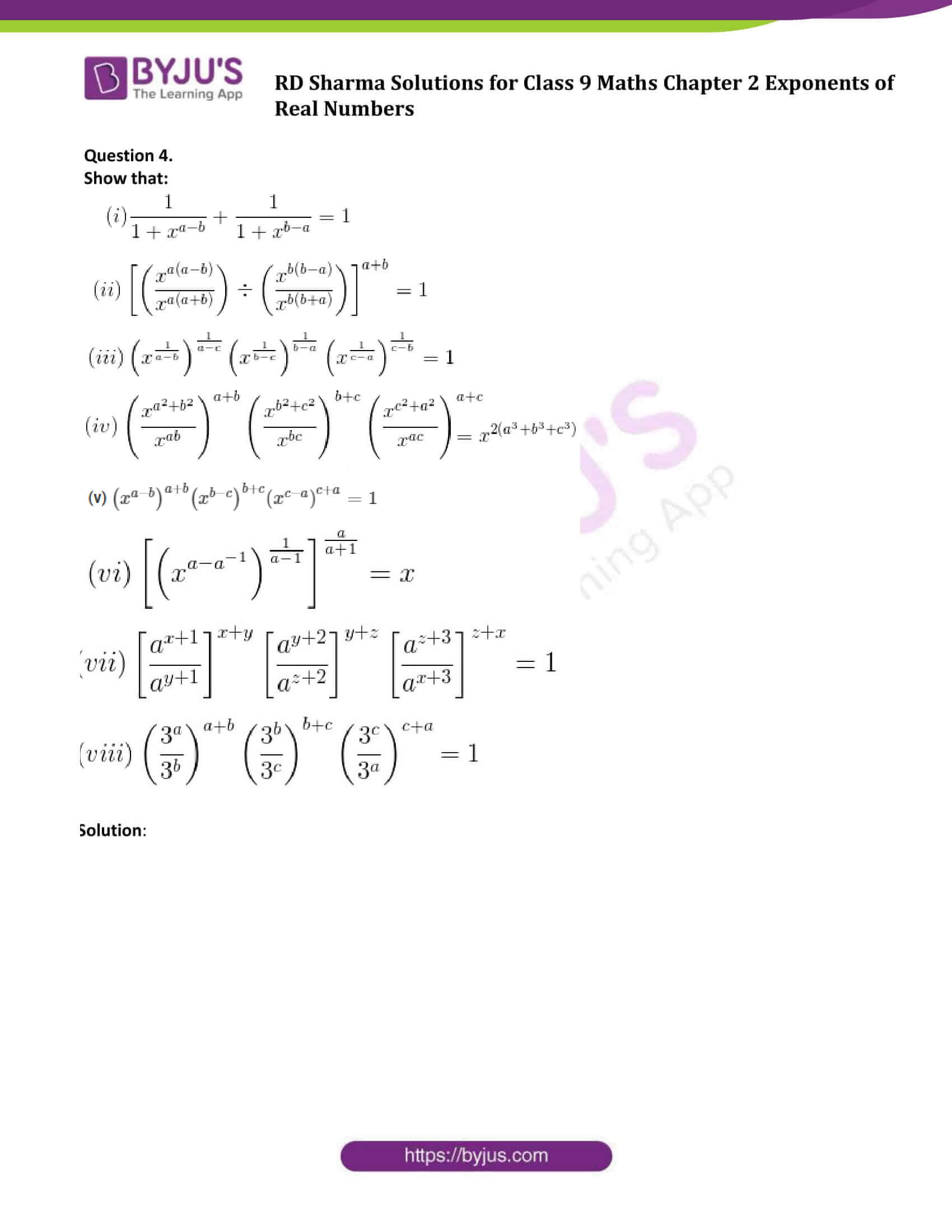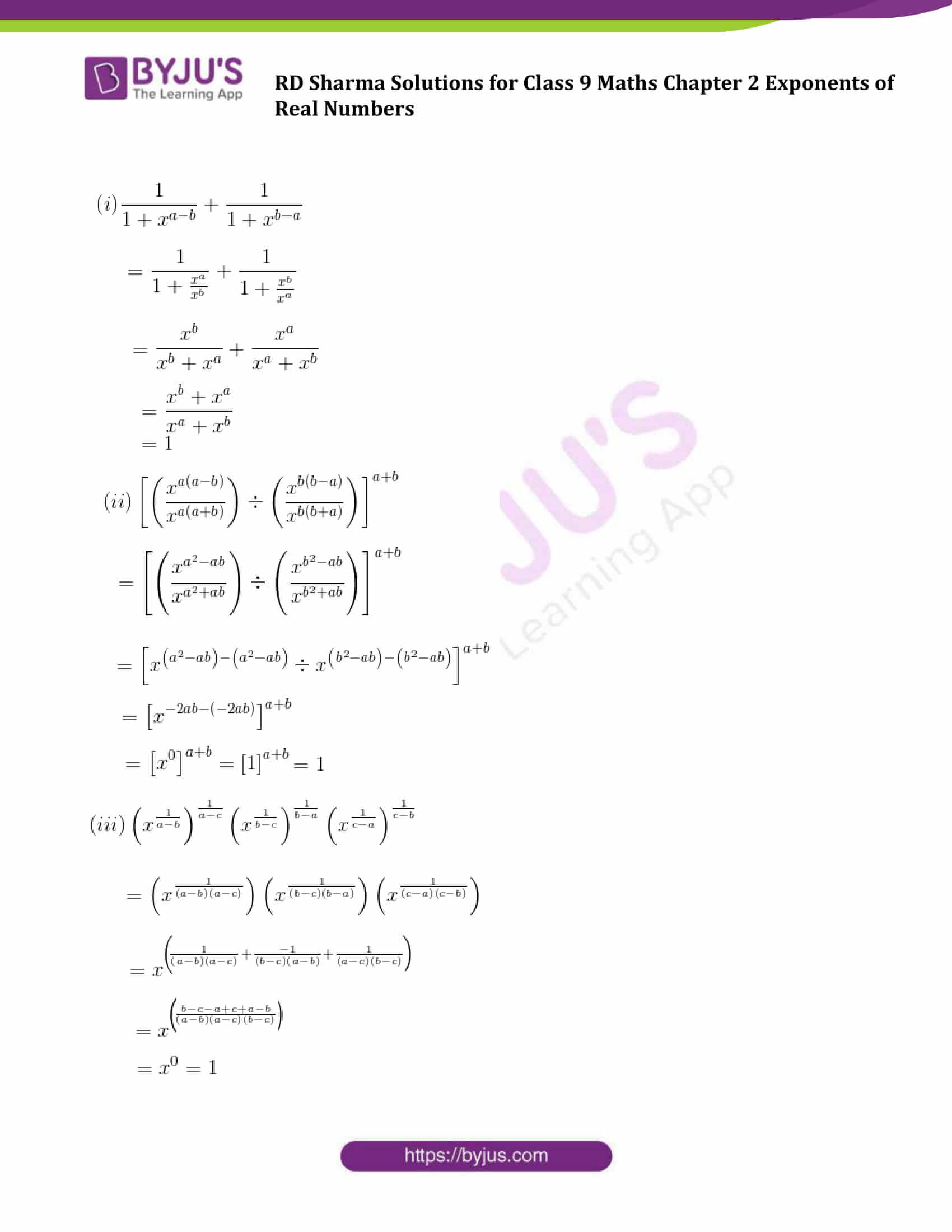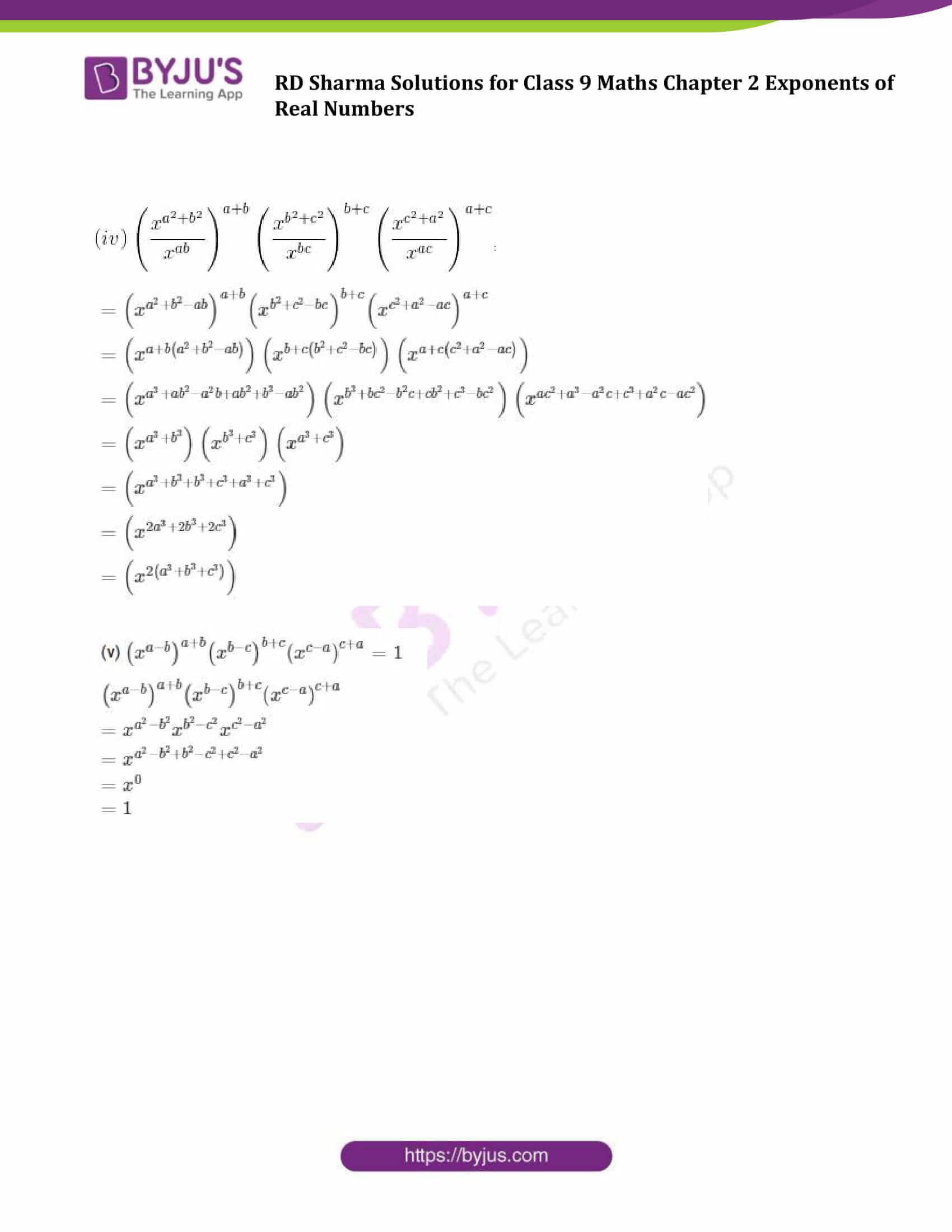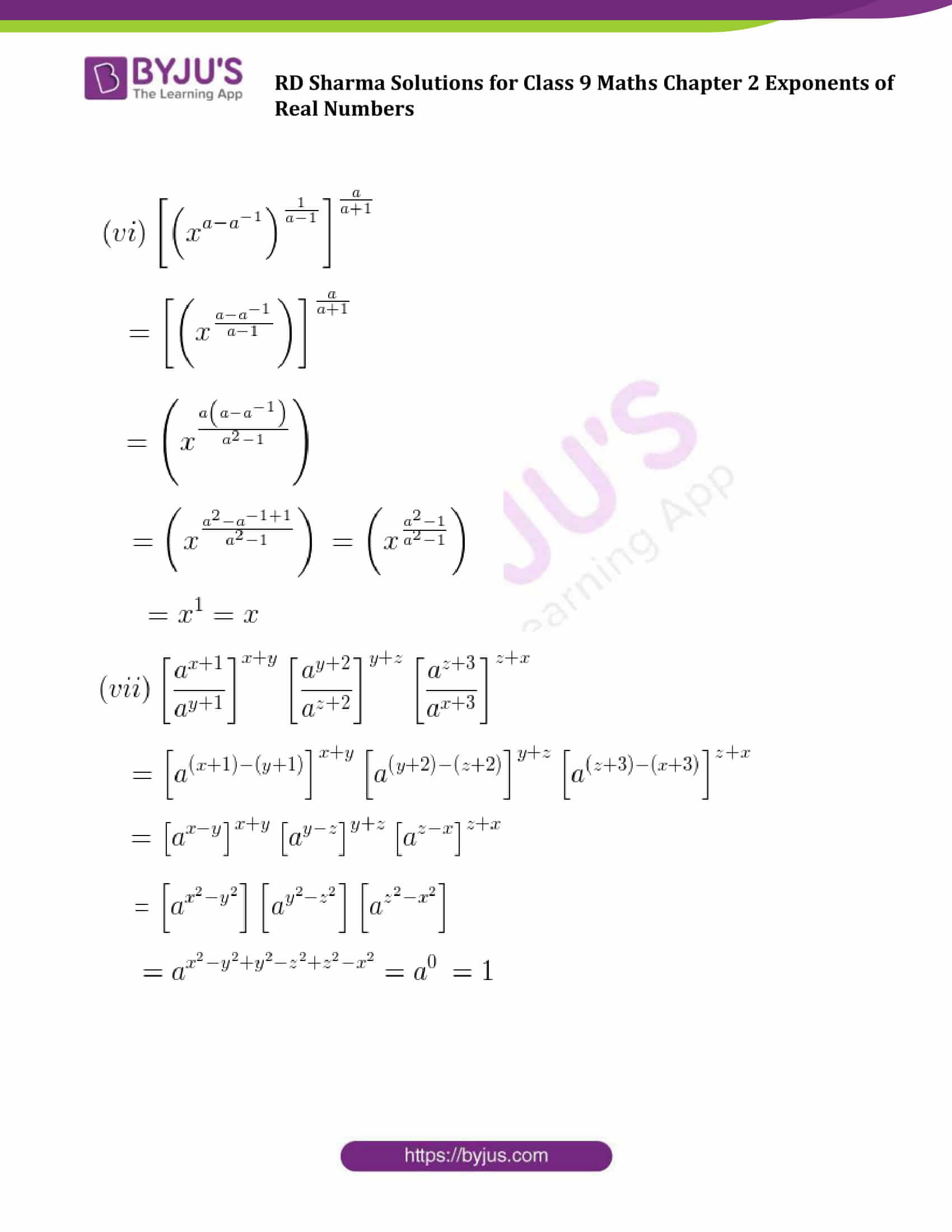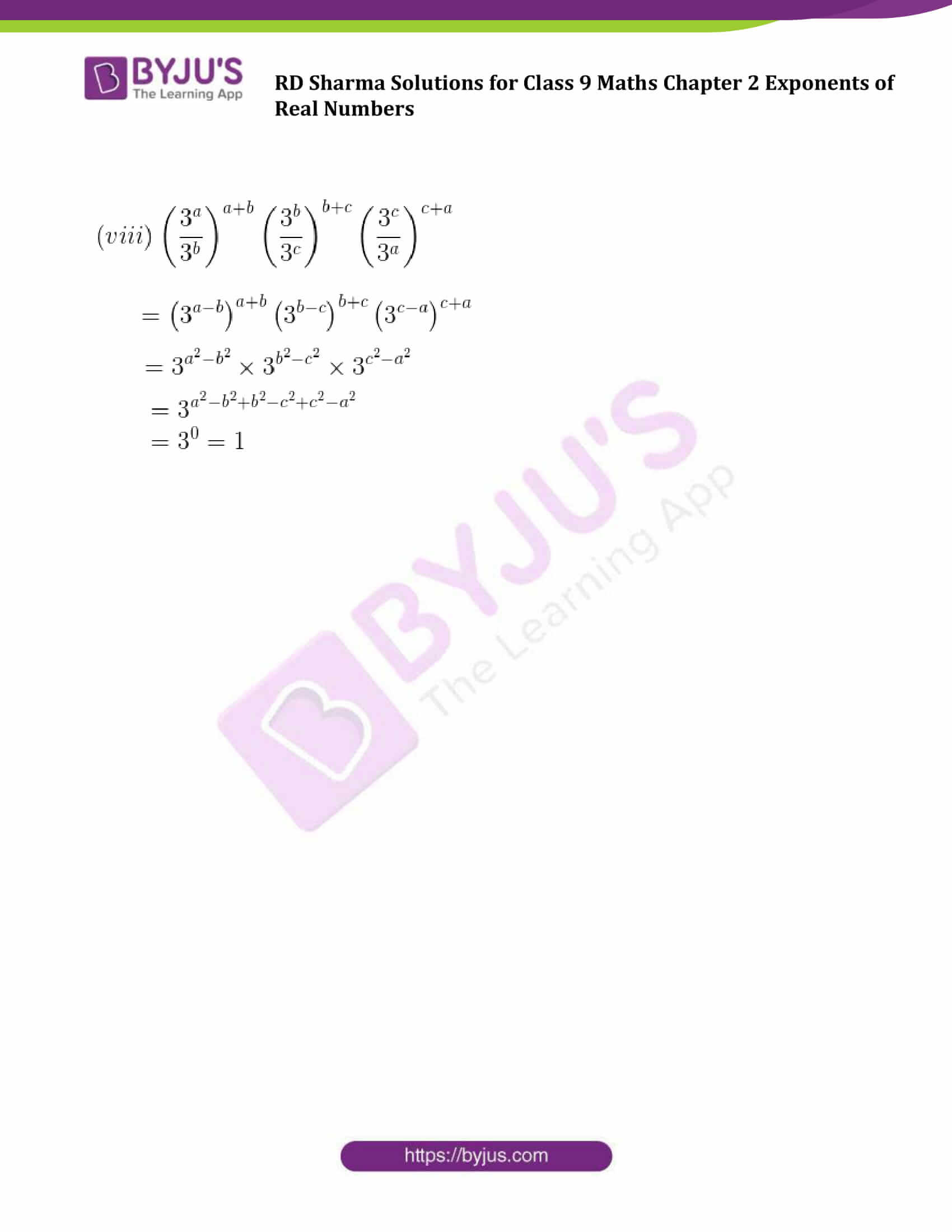### Access Answers to Maths RD Sharma Solutions for Class 9 Chapter 2 Exponents of Real Numbers Exercise 2.2 Page number 2.24

Question 1: Assuming that x, y, z are positive real numbers, simplify each of the following: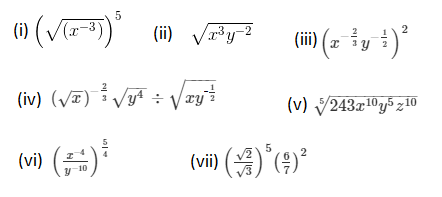Solution: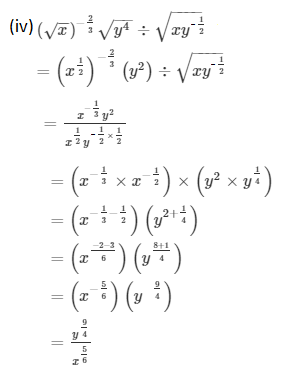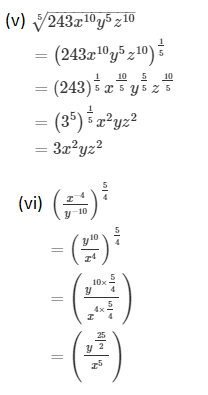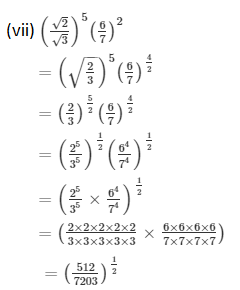Question 2: Simplify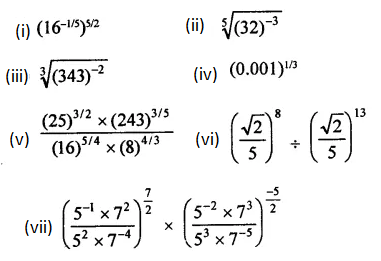Solution: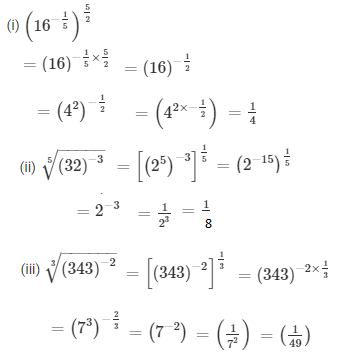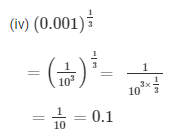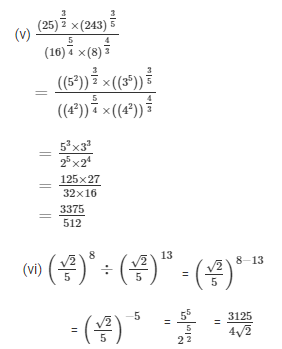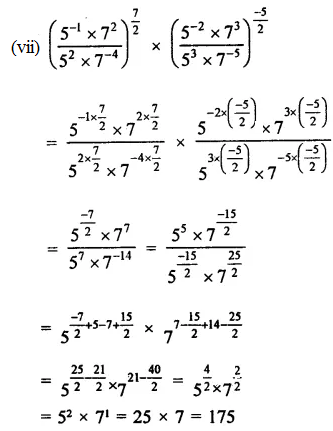Question 3: Prove that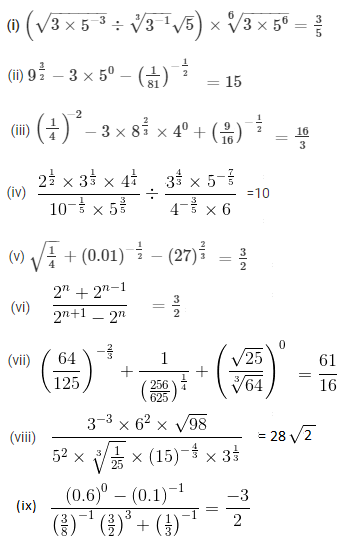Solution:

(i) L.H.S.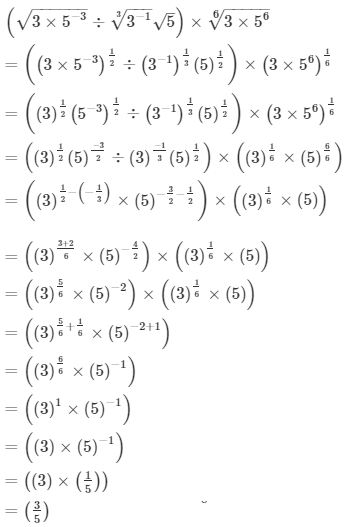=R.H.S.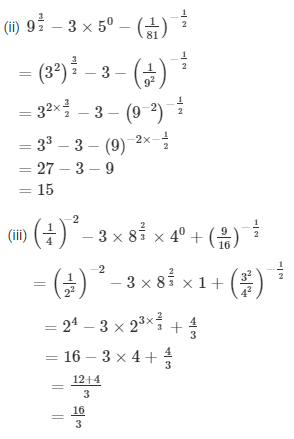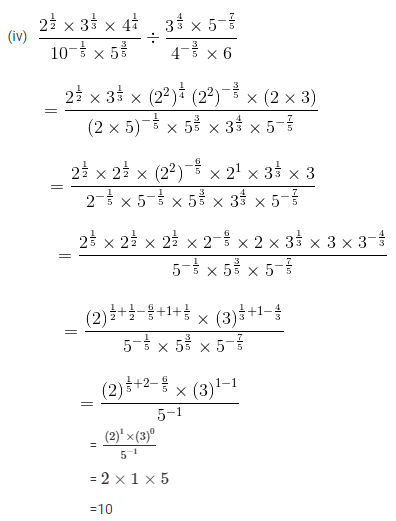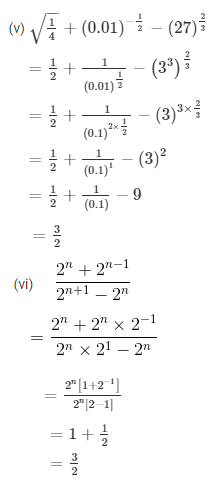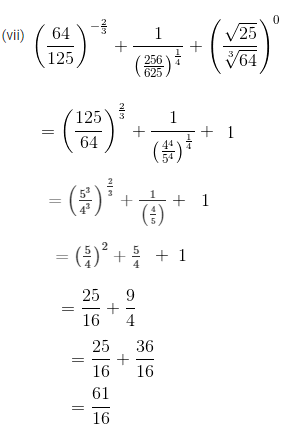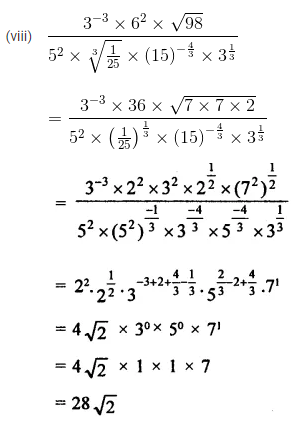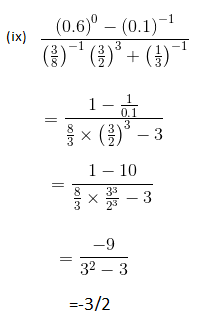Question 4.

Show that: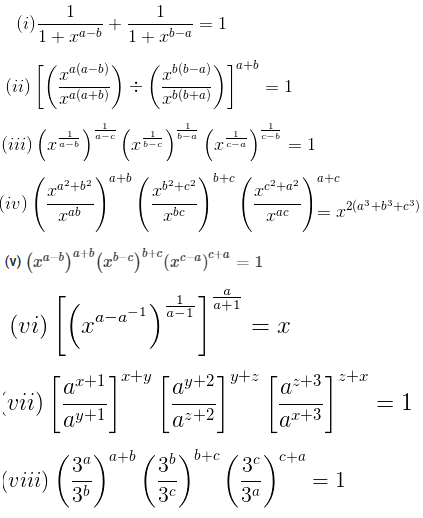Solution: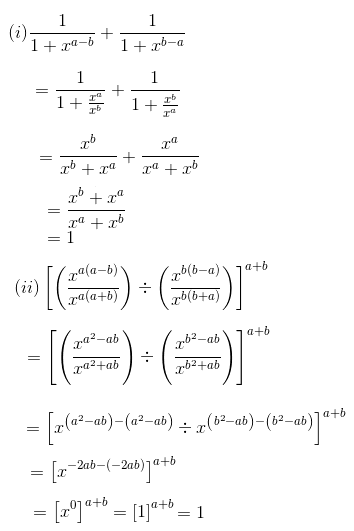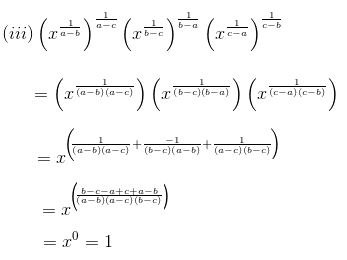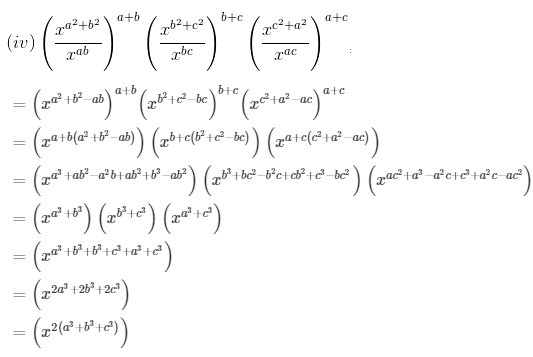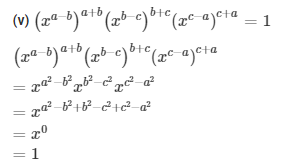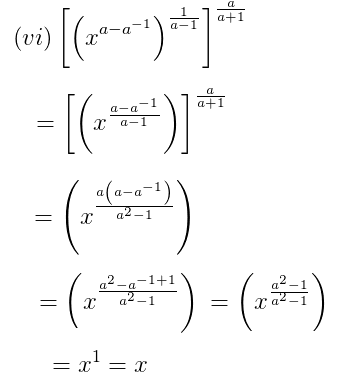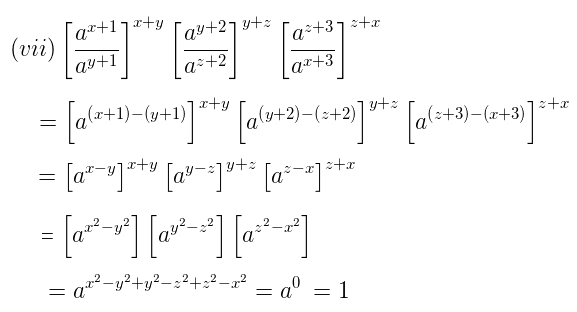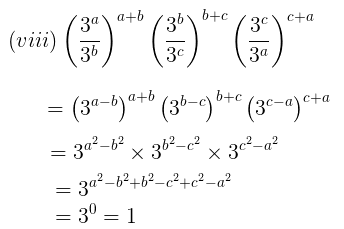## RD Sharma Solutions for Class 9 Maths Chapter 2 Exponents of Real Numbers Exercise 2.2

RD Sharma Solutions Class 9 Maths Chapter 2 Exponents of Real Numbers Exercise 2.2 is based on following topics:

• Rational Exponents of Real Number
• nth root of a positive real number
• Rational Powers
• Laws of rational exponents